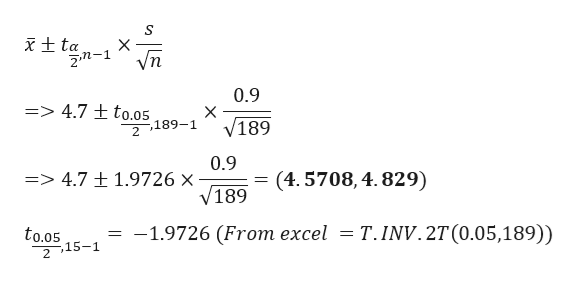# An investigator wants to determine if a new program aimed at reducing the overall number of pregnancies in a highly populated area is effective (reducing average births below 6 per household). In order to carry out the investigation, the investigator conducts a survey of 189 participants in the program and finds the average number of births is 4.7 with a sample standard deviation of 0.9 births. Construct a 95% confidence interval for the average number of births per household in this study. Provide a correct interpretation of your interval.

Question
1 views

An investigator wants to determine if a new program aimed at reducing the overall number of pregnancies in a highly populated area is effective (reducing average births below 6 per household). In order to carry out the investigation, the investigator conducts a survey of 189 participants in the program and finds the average number of births is 4.7 with a sample standard deviation of 0.9 births. Construct a 95% confidence interval for the average number of births per household in this study. Provide a correct interpretation of your interval.

check_circle

Step 1

Given data
Hypothesized mean = 6
Sample Standard deviation = 0.9
Sample mean = 4.7
Sample size n = 189
Formulation of hypothesis
95% confid...help_outlineImage Transcriptioncloseta 2.m-1 0.9 X V189 => 4.7 ± to.05 -,189-1 2 0.9 (4. 5708, 4.829) => 4.7 1.9726 x V189 = -1.9726 (From excel = T. INV. 2T(0.05,189)) to.05 2 15-1 X fullscreen

### Want to see the full answer?

See Solution

#### Want to see this answer and more?

Solutions are written by subject experts who are available 24/7. Questions are typically answered within 1 hour.*

See Solution
*Response times may vary by subject and question.
Tagged in

### Hypothesis Testing